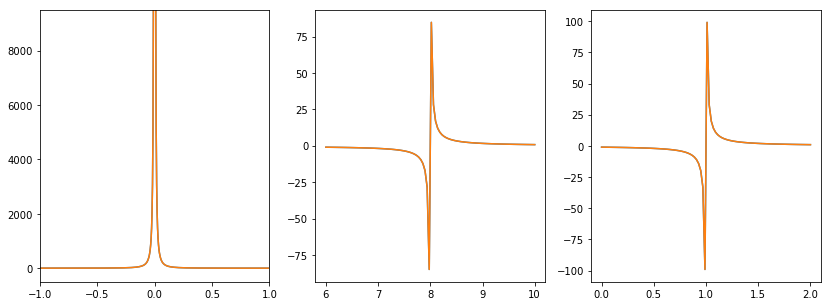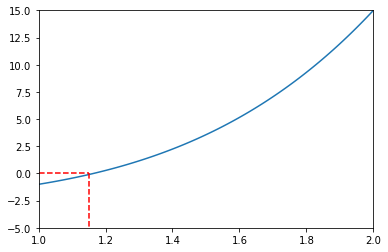## Continuous Functions

A function is said to be continuous at a point $a$ if the following statements hold:

• the function $f$ is defined at $a$
• the limit $\lim_{x \to a} \space f(x)$ exists
• the limit is equal to $f(a)$, $\lim_{x \to a} \space f(x) = f(a)$

Continuity of a function can also be expressed more compactly by the statement: $f(x) \to f(a) \space \text{as} \space f \to a$

Some examples of functions with discontinuity at particular points include:

$$f(x) = \frac{1}{x^2}, \qquad f(x) = \frac{x + 4}{(x - 1)(x - 8)}, \qquad f(x) = \frac{1}{x - 1}$$

We can plot these functions to get a better understanding of where the discontinuity lay.

In :
import numpy as np
import matplotlib.pyplot as plt

In :
def f1(x):
return 1.0 / x ** 2.0

def f2(x):
return (x + 4.0) / ((x - 1.0) * (x - 8.0))

def f3(x):
return 1 / (x - 1)

fx1 = np.linspace(-2, 2, 1000)
fx2 = np.linspace(6, 10, 100)
fx3 = np.linspace(0, 2, 100)

plt.figure(figsize=(14,5))
plt.subplot(131)
plt.plot(fx1, f1(x1), fx1, f1(fx1))
plt.xlim([-1, 1])
plt.ylim([-500, 9500])

plt.subplot(132)
plt.plot(fx2, f2(fx2), fx2, f2(fx2))

plt.subplot(133)
plt.plot(fx3, f3(fx3), fx3, f3(fx3))

plt.show()Although matplotlib plots the line without any breaks, we see each graph has a point where the lines blow up and quickly approaches infinity in either the positive or negative direction.

Interestingly, and somewhat confusingly, although the functions above have a point of discontinuity, they are still said to be continuous functions. The key to this difference is that a function must be defined for all values of $a$. Because the functions cannot be defined at particular values of $x$ due to dividing by $0$, the functions are still continuous.

All polynomials are continuous anywhere in range $(-\infty, \infty)$. Also, any rational function, which has the form $\frac{P(x)}{Q(x)}$ is continuous everywhere except for when $Q(x) = 0$.

Knowing the continuity of a function helps evaluate limits much quicker. For example, consider the function:

$$\lim_{x \to 4} \space \frac{5 + \sqrt{x}}{\sqrt{5 + x}}$$

The function is rational, thus we know it is continuous everywhere except when $Q(x) = 0$. Thus, $x \neq -5$ and we can solve the limit directly:

$$\lim_{x \to 4} \space \frac{5 + \sqrt{x}}{\sqrt{5 + x}} = \lim_{x \to 4} \space f(x) = f(4)$$
$$= \frac{5 + \sqrt{4}}{\sqrt{5 + 4}} = \frac{7}{3}$$

## Intermediate Value Theorem¶

The Intermediate Value Theorem states that if a function $f$ is continuous in a closed interval $[a, b]$ and $n$ is a number between $f(a)$ and $f(b)$ (where $f(a) \neq f(b)$), then there exists a number $c$ in the interval $(a, b)$ such that $f(c) = n$.

The Intermediate Value Theorem can be applied when locating the root of an equation. For example, we can locate the root of the following equation within the interval $[1, 2]$ (should one exist).

$$x^4 + x - 3 = 0$$

Plotting the function first can help us determine if there is a root in the given interval.

In :
def f4(x):
return x ** 4 + x - 3

xvals = np.linspace(1, 2, 100)
y1 = (0, 0)

plt.plot(xvals, f4(xvals))
plt.plot((0, 1.15), (0, 0), 'r--')
plt.plot((1.15, 1.15), (0,-5), 'r--')

plt.xlim([1, 2])
plt.ylim([-5, 15])
plt.show()It appears the equation does have a root located in the interval $[1, 2]$. We can also show this with the Intermediate Value Theorem, we take $a = 1$, $b = 2$ and $n = 0$:

$$f(1) = (1)^4 + (1) - 3 = -1 < 0$$
$$f(2) = (2)^4 + (1) - 3 = 14 > 0$$

Therefore, $f(1) < 0 < f(2)$ and $n$ is a number between $f(1)$ and $f(2)$. We can get a more accurate interval of where the root is located by setting a tighter interval around the location of the root and applying the Intermediate Value Theorem. Judging by the graph, the root appears to be located in the interval $[1.1, 1.2]$, which we can verify by setting $a = 1.1$, $b = 1.2$ and $n = 0$.

In :
(f4(1.1), f4(1.2))

Out:
(-0.4358999999999993, 0.27360000000000007)

Thus, we now know the root is located in the interval $[-0.44, 0.27]$.